## Cubes and Dices

#### Cubes and Dices

1. How many cubes are there in below figure?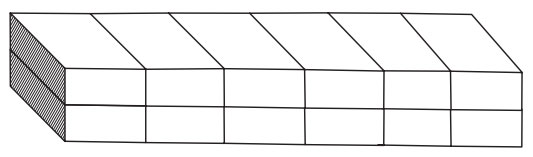1. There is 6 columns with 2 cubes each.

##### Correct Option: C

There is 6 columns with 2 cubes each.
Total boxes = 6 × 2 = 12

1. How many cubes are there in below figure?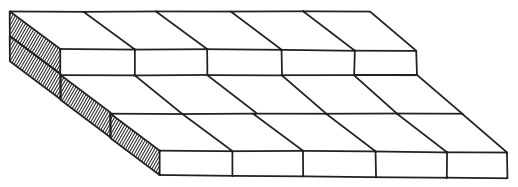1. There are total 4 rows and 5 boxes in each row.

##### Correct Option: A

There are total 4 rows and 5 boxes in each row,
then 5 × 4 = 20 boxes.

1. How many cubes are there in below figure?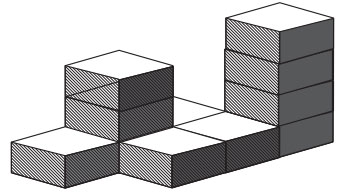1. 4 columns with 1 cubes = 4 cubes
Similarly calculate the others also.

##### Correct Option: B

4 columns with 1 cubes = 4 cubes
1 columns with 3 cubes = 3
1 columns with 4 cubes = 4
Total cubes = 4 + 3 + 4 = 11

1. Two Position of a dice are shown below as (i) and (i). When six is at the bottom, what number will be at the top?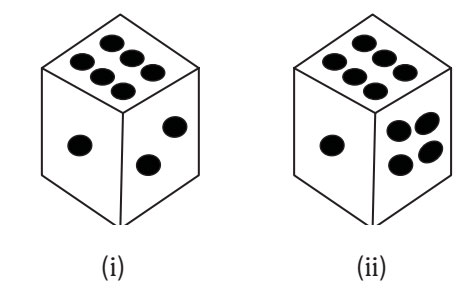1. From both the figures we find that numbers 1,2 and 4 dots appear adjacent to 6.
For the better understanding take a dice and apply the same steps which is mentioned above.

##### Correct Option: D

From both the figures we find that numbers 1,2 and 4 dots appear adjacent to 6. thus, the number 5 dots will appear opposite to 6. Therefore when 6 is at the bottom, then 5 will be at the top.

1. Two positions of a dice are shown below (i) and (ii). When 1 is at the top. which number will be at the bottom?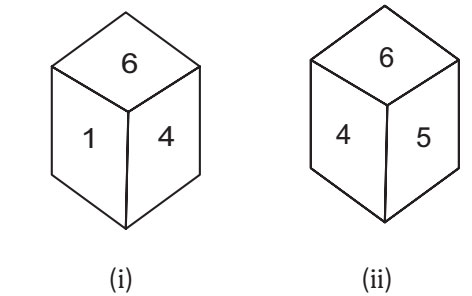1. as we can observe the position of 6 is same in both the figures and position of 4 moves from Right hand side to Left hand side (clock wise direction).
For the better understanding take a dice and apply the same steps which is mentioned above.

##### Correct Option: C

as we can observe the position of 6 is same in both the figures and position of 4 moves from Right hand side to Left hand side (clock wise direction).